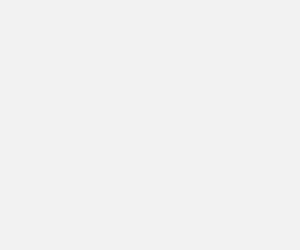×The Free 30-Day CAT RC Course

"It is designed to help you excel in the upcoming CAT 2023 exam"

-By Lavleen Kaur Kapoor. Over 1,73,000 Subscribers

No thanks >

OPEN

## IBSAT, CAT, XAT, NMAT, GMAT

OPEN#### Get Personalized MBA Mentorship

Connect with my team on WhatsApp for unbiased MBA College Selection advice.

# XAT 2023: How to solve Data Interpretation Questions?

XAT Exam Date 8th January 2023MBA aspirants who are appearing in XAT 2023 in January,2023  may find following questions with solutions very useful:

The following graphs shows the revenue (in \$ million) of three companies in their initial six years of operations, in an economy which is characterized by a persistent inflation.Question 1)

In 2010, which could be a valid statement about the revenues (adjusted for inflation) of these three companies?
1. Revenues of all three companies were equal.
2. Revenues of all three companies could be equal.
3. Revenue of Yahoo was definitely less than Facebook which was definitely less than Google.
4. Total of Yahoo and Facebook was definitely higher than that of Google
5. None of the above
Solution:

Option 5. None of the above

Explanation:
No data has been given in the question as to which year amongst the given years in the graph is 2010. Hence this question is incorrect.

Note:  By trial and error method, let us assume all the years one by one to be 2010.
• Revenues  of  all  the  three  companies  are  not  equal  in any  of  the  6  years  and  hence  option  A  and  B  are incorrect.
• Revenues  of  Google  were  less  than  that  of  Facebook and  Yahoo  in  the  first  three  years  mentioned  in  the graph and hence option C is also incorrect
• Total  of  Yahoo  and  Facebook  was  less  than  Google  in the  fifth  year  shown  in  the  graph  and  so  option  D  is also wrong.
• If  this  question  needs  to  be  compulsorily  answered, then  option  E,  none  of  the  above  would  be  the  correct one
• However this doesn’t refute the fact that this question is  an  incorrect  one  as  data  about  the  regarding  which year represents  2010 is not given.
• Hence, Option 5
Question 2)

The difference in the average percentage increase in revenues, from 4th to 6th year, of Yahoo and Facebook is:
1. 35%
2. 40%
3. 45%
4. 50%
5. 55%
Solution:

Option 1. 35%

Explanation:
• Revenue of Yahoo in 2006 = 1200
• Revenue of Yahoo in 2004 = 250
• So, percentage increase = (950/250) × 100 = 380
• Average percentage increase = 380/2 = 190
• Revenue of Facebook in 2006 = 2000
• Revenue of Facebook in 2004 = 350
• So, percentage increase = (1650/350) × 100 = 235.71
• So, percentage difference in both the values = [(235.71 – 190)/190] × 100 = 24.05
• None of the options are less than 35%, so the question is incorrect.
• However “None of the above” is not one of the given options and hence we will have to take the option closest to 24.05 as the correct answer.
• Hence, Option 1.
Question 3)

What would have been Facebook’s revenue (in \$ million)  in  its  sixth  year  of  operation  if the company had matched Google’s percentage growth  in  revenues  from  the  fifth  to  the sixth year?

Choose the option that is nearest to the answer.
1. 1600
2. 1700
3. 1900
4. 2100
5. None of the above
Solution:

Option 1. 1600

Explanation:
• Percentage increase in Google’s growth between 5th and 6th year = (3250 – 1500)/1500 × 100 = 116.67
• So, required value of Facebook revenue after 6th year = 750 × 216.67 = 1625.05
• So, the closest answer is 1600.
• Hence, Option 1.
For Further more details / syllabus on XAT 2023, please visit

Stay ahead and Stay inspired with MBA Rendezvous